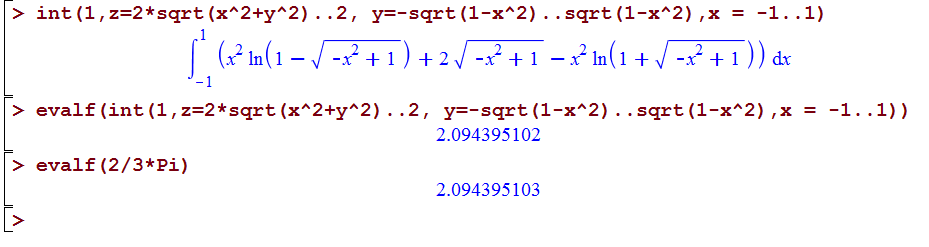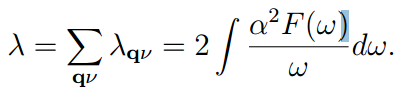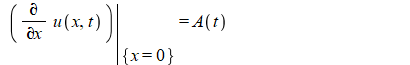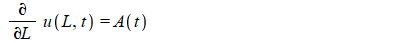## Matrix coefficients of a list of polynomial w.r.t....

Let us consider L be the following list of 6 lists of polynomials which all of their polynomials are linear combination of B=[x^2,x*y,z^2,1].

L:=[[a*x^2+b*x*y-1, -(a*b-b)*x*y/a-z^2+(a-1)/a, -a*c*z^2/(b*(a-1))+(b+c)/b], [a*x^2+b*x*y-1, -(a*b-b)*x*y/a-z^2+(a-1)/a, 1],

[a*x^2+b*x*y-1, -z^2+(a-1)/a, c*x*y+1], [b*x*y-1, -x^2-z^2, (b+c)/b], [-1, -x^2-z^2, c*x*y], [-1, -x^2-z^2]].

Now, I need the matrix coefficients of any member of L (please note that any matrix has 4 columns according to the list B) . Is there any command for this?

## help with understanding modules...

Ok so when a module is loaded, there is a .dll file that loads a list of symbols into maple.exe, what can be done if an error occurs for which the debugging output declares that the PDB file for the module could not be found?

I have read that the program database file stores debugging information related to the locating symbols, how can i fix this if my computer isn't finding theres files for a list of .dll it attempts to load into maple.exe, or is there a way for which i can make these files for each corresponding module?

## Show that an ODE has an eighth dimensional lie sym...

u(vu)+ uvvuu - 2vvu = 0

using maple show that the ode has an eighth dimensional lie symmetry group

## Why is this triple integral not evaluating....

For some reason the Maple software is not evaluating the last bounds for a triple integral.

But the evalf command works.## Why does Maple 2018.1 want me to upgrade to Maple ...

I have Maple through the school where I teach math, and the IT-guy gave us Maple 2018.1 to install.

But after a while even though I have Maple 2018.1 installed on my computer Windows 10 pc, then Maple 2018.1 surgests that I upgrade to 2018.1?? Any idea why?## split a polynomial based on leading integer coeffi...

I could not find a command that splits a polynomial in parts based on leading integer coefficients, so I wrote a procedure. It works well, but I still wonder if there is no simpler way of doing this?
My aim is to investigate whether there is some way of factoring this and other polynomials.

kind regards,
Harry Garst>>(1)
 >(2)
 >(3)
 >(4)
 >(5)
 >(6)
 >(7)
 >(8)
 >(9)
 >(10)
 >(11)
 >(12)## How to perform numerical integration with set of v...

Dear all,

Greeting!

I am a new user of Maple. I want to perform a simple (to me, it is very difficult) integration  defined as followsrange from 0 to omega (frequency). alpha^2F (omega) is constant function with set of values (already calculated) for the corresponding frequency. I have calculated the frequencies ranging from 54 to 900 cm-1. Suppose I have alpha^2F(omega)=0, 0.5,0.6,0.7......0.1 for omega=58, 80, 110, 190,.....800, how can I perform this integration  in Maple? Please help me how can I cope with this problem.

Thanks a lot.

Best Regards,

## Maple hangs in pdsolve on a standard PDE...

Trying Maple on a textbook problem to verify my hand solution.

But Maple pdsolve hangs with the mserver.exe tallomg almost 100% CPU and over 10 GB of RAM!

I waited for almost 20 minutes. Tried another time, same thing.

It is no problem if Maple can't solve this, but Maple seems to suffer from too many hangs when it is not able to solve a problem. I've had similar problems with dsolve also.

This is on windows 10, 64 bit with Maple 2018.2 With Physics updates version 218

```restart;
```

218

```interface(showassumed=0);
infolevel[pdsolve]:=2;
pde :=  diff(u(x,t),t)=k*diff(u(x,t),x\$2)+Q(x,t);
ic  :=  u(x,0)=f(x);
bc  :=  eval(diff(u(x,t),x),x=0)=A(t),eval(diff(u(x,t),x),x=1)=B(t);
sol:=pdsolve({pde,ic,bc},u(x,t)) assuming t>0,k>0;
```

then

```
* trying method "_Fn" for 2nd order PDEs
-> trying "linear_in_xt"
-> trying "BC_equal_0"
* trying method "_Cn_cn" for 2nd order PDEs
Trying travelling wave solutions as power series in tanh ...
Trying travelling wave solutions as power series in ln ...
Trying travelling wave solutions as power series in tanh ...
Trying travelling wave solutions as power series in ln ...
* trying method "Wave" for 2nd order PDEs
-> trying "Cauchy"
-> trying "SemiInfiniteDomain"
-> trying "WithSourceTerm"
* trying method "Heat" for 2nd order PDEs
-> trying "SemiInfiniteDomain"
-> trying "WithSourceTerm"
* trying method "Series" for 2nd order PDEs
-> trying "ThreeBCsincos"
-> trying "FourBC"
-> trying "ThreeBC"
-> trying "ThreeBCPeriodic"
-> trying "WithSourceTerm"
* trying method "_Fn" for 2nd order PDEs
-> trying "linear_in_xt"
-> trying "BC_equal_0"
* trying method "_Cn_cn" for 2nd order PDEs```

And here is hangs. Notice that because both ends are Neumann, there is no unique solution to this problem.  So the solution will contain arbitrary constant. May be this is what made pdsolve hang?

No it is not. Trying with only one end nonhomogeneous  Neumann, and the other end Dirichlet, it still hangs. The problem seems to be with one end is nonhomogeneous  Neumann, which is a function of time. So this hangs also (same place)

```restart;
interface(showassumed=0);
infolevel[pdsolve]:=2;
pde :=  diff(u(x,t),t)=k*diff(u(x,t),x\$2)+Q(x,t);
ic  :=  u(x,0)=f(x);
bc  :=  eval(diff(u(x,t),x),x=0)=A(t),eval(diff(u(x,t),x),x=1)=0;
sol:=pdsolve({pde,ic,bc},u(x,t)) assuming t>0,k>0;```

And this also

```restart;
interface(showassumed=0);
infolevel[pdsolve]:=2;
pde :=  diff(u(x,t),t)=k*diff(u(x,t),x\$2)+Q(x,t);
ic  :=  u(x,0)=f(x);
bc  :=  eval(diff(u(x,t),x),x=0)=sin(t),eval(diff(u(x,t),x),x=1)=0;
sol:=pdsolve({pde,ic,bc},u(x,t)) assuming t>0,k>0;```

But this does not hang

```interface(showassumed=0);
infolevel[pdsolve]:=2;
pde :=  diff(u(x,t),t)=k*diff(u(x,t),x\$2)+Q(x,t);
ic  :=  u(x,0)=f(x);
bc  :=  eval(diff(u(x,t),x),x=0)=1,eval(u(x,t),x=1)=0;
sol:=pdsolve({pde,ic,bc},u(x,t)) assuming t>0,k>0;
```

The issue seems to be when one end is nonhomogeneous  Neumann which is function of time.

Is there a workaround so it does not hang? The complaint is that Maple hangs, and not that Maple unable to solve the PDE.

## Calculating values of function of solutions of ODE...

```restart;
#PDE
PDE := (diff(u(x,y), x,x))-1/x*(diff(u(x, y), x)) -x^2*(diff(u(x,y), y,y))= 2;
BCs:=u(0,t) = 0, u(1,t) = 0;

ICs:=u(x,0) =0, D(u)(x,0)=2 ;
num_sol := pdsolve(PDE, {BCs,ICs}, numeric);
BCs := u(0, t) = 0, u(1, t) = 0;
ICs := u(x, 0) = 0, D(u)(x, 0) = 2;
num_sol := pdsolve(PDE, {BCs,ICs}, numeric);

#ODE
ODE:=diff(y(x),x,x)+16*diff(y(x),x)=0;
BCs:=y(0) =0, y(2)=3 ;
ode_num_sol:=dsolve({ODE,BCs},numeric);
```

Suppose that we solved numerically an ODE or PDE like above.
How to calculate values of function y or u at special values x like analytical solutions?

For example; u(1,1)=? y(1)=? etc.

the_code.mw

## on the syntax of eval(diff(u(x,t),x=0)..... ...

Why

```eval(diff(u(x,t),x),x=0)=A(t)
```

givesBut

```eval(diff(u(x,t),x),x=L)=A(t)
```

givesI was expecting the same syntax in both cases. It seems for numbers Maple uses the first syntax and for symbols it uses the second syntax.

## What is the mathematical description of a velodrom...

I would like to animate the motion of a bicycle racer on a classic velodrome track i.e. one with varied vertical and horizontal curvatures along its length.

Is there a source which explains the math expressions which model the shape of such a track?

## Inverse List !!...

Let us consider S := [x^2 = A1, x*y = A2, z^2 = A3] as a list. Is there any command to obtain  SS = [A1=x^2 , A2=x*y , A3=z^2] from S?

## Forum activity ...

It seems that the forum activity of the user     is no longer accessible.
Is it a site problem?

## How can one decide if a vector is a positive linea...

My goal is to find a positive linear combination of a vector vec over a set of vectors M1,...,M6. In this case the Mi are the columns of the matrix M.

My reasoning is: solve the linear system M.x=vec, which gives me a parametrized solution, then check if there is at least one positive solution.

M:=Matrix([[3, 0, 2, 2, 1, 1], [-1, -1, 0, -1, 0, -1], [0, 3, 0, 1, 1, 2], [3, 0, 1, 2, 0, 1], [-1, -1, -1, -1, -1, -1]]);

vec:= Vector[column](5, [3, 1, 0, 0, -2]);

x:=LinearSolve(M,vec, free = s);But when I use the LinearMultivariateSystem function I get an error

LinearMultivariateSystem({x>=0, x>=0, x>=0, x>=0, x>=0, x>=0},[s,s,s, s, s,s]);
Error, (in Utilities:-SimpleAnd) invalid input: a string/name list is expected for sort method `lexorder`

Can anyone tell me what is wrong in the code?

If I manually type in the expressions, and use variables such as x,y,z... then I get the solution, but I want to put the function into a loop.

I am very open to suggestions on how to decide if a vector is a positive linear combination of other vectors.

Maria